Introduction to Measurements & Error Analysis

The Uncertainty of Measurements

Some numerical statements are exact: Mary has 3 brothers, and 2 + 2 = 4. However, all measurements have some degree of uncertainty that may come from a variety of sources. The process of evaluating this uncertainty associated with a measurement result is often called uncertainty analysis or error analysis.

The complete statement of a measured value should include an estimate of the level of confidence associated with the value. Properly reporting an experimental result along with its uncertainty allows other people to make judgements about the quality of the experiment, and it facilitates meaningful comparisons with other similar values or a theoretical prediction. Without an uncertainty estimate, it is impossible to answer the basic scientific question: "Does my result agree with a theoretical prediction or results from other experiments?" This question is fundamental for deciding if a scientific hypothesis is confirmed or refuted.

When we make a measurement, we generally assume that some exact or true value exists based on how we define what is being measured. While we may never know this true value exactly, we attempt to find this ideal quantity to the best of our ability with the time and resources available. As we make measurements by different methods, or even when making multiple measurements using the same method, we may obtain slightly different results. So how do we report our findings for our best estimate of this elusive true value? The most common way to show the range of values that we believe includes the true value is:

measurement = best estimate ± uncertainty

Lets take an example. Suppose you want to find the mass of a gold ring that you would like to sell to a friend. You do not want to jeopardize your friendship, so you want to get an accurate mass of the ring in order to charge a fair market price. By simply examining the ring in your hand, you estimate the mass to be between 10 and 20 grams, but this is not a very precise estimate. After some searching, you find an electronic balance which gives a mass reading of 17.43 grams. While this measurement is much more precise than the original estimate, how do you know that it is accurate, and how confident are you that this measurement represents the true value of the rings mass? Since the digital display of the balance is limited to 2 decimal places, you could report the mass as m = 17.43 ± 0.01 g. Suppose you use the same electronic balance and obtain several more readings: 17.46 g, 17.42 g, 17.44 g, so that the average mass appears to be in the range of 17.44 ± 0.02 g. By now you may feel confident that you know the mass of this ring to the nearest hundreth of a gram, but how do you know that the true value definitely lies between 17.43 g and 17.45 g? Since you want to be honest, you decide to use another balance which gives a reading of 17.22 g. This value is clearly below the range of values found on the first balance, and under normal circumstances, you might not care, but you want to be fair to your friend. So what do you do now?

To help answer these questions, we should first define the terms accuracy and precision:

Accuracy is the closeness of agreement between a measured value and a true or accepted value. Measurement error is the amount of inaccuracy.

Precision is a measure of how well a result can be determined (without reference to a theoretical or true value). It is the degree of consistency and agreement among independent measurements of the same quantity; also the reliability or reproducibility of the result.

The statement of uncertainty associated with a measurement should include factors that affect both the accuracy and precision of the measurement.

Caution:  Unfortunately the terms error and uncertainty are often used interchangeably to describe both imprecision and inaccuracy. This usage is so common that it is impossible to avoid entirely. Whenever you encounter these terms, make sure you understand whether they refer to accuracy or precision, or both.

Notice that in order to determine the accuracy of a particular measurement, we have to know the ideal, true value, which we really never do. Sometimes we have a "textbook" measured value which is known precisely, and we assume that this is our "ideal" value, and use it to estimate the accuracy of our result. Other times we know a theoretical value which is calculated from basic principles, and this also may be taken as an "ideal" value. But physics is an empirical science, which means that the theory must be validated by experiment, and not the other way around. We can escape these difficulties and retain a useful definition of accuracy by assuming that, even when we do not know the true value, we can rely on the best available accepted value with which to compare our experimental value.

For our example with the gold ring, there is no accepted value with which to compare, and both measured values have the same precision, so we have no reason to believe one more than the other. The only way to assess the accuracy of the measurement is to compare with a known standard. For this situation, it may be possible to calibrate the balances with a standard mass that is accurate within a narrow tolerance and is traceable to a primary mass standard at the National Institute of Standards and Technology (NIST). Calibrating the balances should eliminate the discrepancy between the readings and provide a more accurate mass measurement.

Precision is often reported quantitatively by using relative or fractional uncertainty: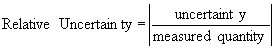(1) For example, m = 75.5 ± 0.5 g has a fractional uncertainty of: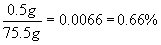Accuracy is often reported quantitatively by using relative error: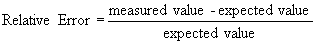(2)

If the expected value for m is 80.0 g, then the relative error is:Note: The minus sign indicates that the measured value is less than the expected value.

When analyzing experimental data, it is important that you understand the difference between precision and accuracy. Precision indicates the quality of the measurement, without any guarantee that the measurement is "correct." Accuracy, on the other hand, assumes that there is an ideal value, and tells how far your answer is from that ideal, "right" answer. These concepts are directly related to random and systematic measurement errors.

Types of Errors

Measurement errors may be classified as either random or systematic, depending on how the measurement was obtained (an instrument could cause a random error in one situation and a systematic error in another).

Random errors are statistical fluctuations (in either direction) in the measured data due to the precision limitations of the measurement device. Random errors can be evaluated through statistical analysis and can be reduced by averaging over a large number of observations (see standard error).

Systematic errors are reproducible inaccuracies that are consistently in the same direction. These errors are difficult to detect and cannot be analyzed statistically. If a systematic error is identified when calibrating against a standard, the bias can be reduced by applying a correction or correction factor to compensate for the effect. Unlike random errors, systematic errors cannot be detected or reduced by increasing the number of observations.

Our strategy is to reduce as many sources of error as we can, and then to keep track of those errors that we cant eliminate. It is useful to study the types of errors that may occur, so that we may recognize them when they arise.

Common sources of error in physics laboratory experiments:

Incomplete definition (may be systematic or random) - One reason that it is impossible to make exact measurements is that the measurement is not always clearly defined. For example, if two different people measure the length of the same rope, they would probably get different results because each person may stretch the rope with a different tension. The best way to minimize definition errors is to carefully consider and specify the conditions that could affect the measurement.

Failure to account for a factor (usually systematic)  The most challenging part of designing an experiment is trying to control or account for all possible factors except the one independent variable that is being analyzed. For instance, you may inadvertently ignore air resistance when measuring free-fall acceleration, or you may fail to account for the effect of the Earths magnetic field when measuring the field of a small magnet. The best way to account for these sources of error is to brainstorm with your peers about all the factors that could possibly affect your result. This brainstorm should be done before beginning the experiment so that arrangements can be made to account for the confounding factors before taking data. Sometimes a correction can be applied to a result after taking data to account for an error that was not detected.

Environmental factors (systematic or random) - Be aware of errors introduced by your immediate working environment. You may need to take account for or protect your experiment from vibrations, drafts, changes in temperature, electronic noise or other effects from nearby apparatus.

Instrument resolution (random) - All instruments have finite precision that limits the ability to resolve small measurement differences. For instance, a meter stick cannot distinguish distances to a precision much better than about half of its smallest scale division (0.5 mm in this case). One of the best ways to obtain more precise measurements is to use a null difference method instead of measuring a quantity directly. Null or balance methods involve using instrumentation to measure the difference between two similar quantities, one of which is known very accurately and is adjustable. The adjustable reference quantity is varied until the difference is reduced to zero. The two quantities are then balanced and the magnitude of the unknown quantity can be found by comparison with the reference sample. With this method, problems of source instability are eliminated, and the measuring instrument can be very sensitive and does not even need a scale.

Failure to calibrate or check zero of instrument (systematic) - Whenever possible, the calibration of an instrument should be checked before taking data. If a calibration standard is not available, the accuracy of the instrument should be checked by comparing with another instrument that is at least as precise, or by consulting the technical data provided by the manufacturer. When making a measurement with a micrometer, electronic balance, or an electrical meter, always check the zero reading first. Re-zero the instrument if possible, or measure the displacement of the zero reading from the true zero and correct any measurements accordingly. It is a good idea to check the zero reading throughout the experiment.

Physical variations (random) - It is always wise to obtain multiple measurements over the entire range being investigated. Doing so often reveals variations that might otherwise go undetected. These variations may call for closer examination, or they may be combined to find an average value.

Parallax (systematic or random) - This error can occur whenever there is some distance between the measuring scale and the indicator used to obtain a measurement. If the observer's eye is not squarely aligned with the pointer and scale, the reading may be too high or low (some analog meters have mirrors to help with this alignment).

Instrument drift (systematic) - Most electronic instruments have readings that drift over time. The amount of drift is generally not a concern, but occasionally this source of error can be significant and should be considered.

Lag time and hysteresis (systematic) - Some measuring devices require time to reach equilibrium, and taking a measurement before the instrument is stable will result in a measurement that is generally too low. The most common example is taking temperature readings with a thermometer that has not reached thermal equilibrium with its environment. A similar effect is hysteresis where the instrument readings lag behind and appear to have a "memory" effect as data are taken sequentially moving up or down through a range of values. Hysteresis is most commonly associated with materials that become magnetized when a changing magnetic field is applied.

Personal errors come from carelessness, poor technique, or bias on the part of the experimenter. The experimenter may measure incorrectly, or may use poor technique in taking a measurement, or may introduce a bias into measurements by expecting (and inadvertently forcing) the results to agree with the expected outcome.

Gross personal errors, sometimes called mistakes or blunders, should be avoided and corrected if discovered. As a rule, gross personal errors are excluded from the error analysis discussion because it is generally assumed that the experimental result was obtained by following correct procedures. The term human error should also be avoided in error analysis discussions because it is too general to be useful. Estimating Experimental Uncertainty for a Single Measurement

Any measurement you make will have some uncertainty associated with it, no matter how precise your measuring tool. How do you actually determine the uncertainty, and once you know it, how do you report it?

The uncertainty of a single measurement is limited by the precision and accuracy of the measuring instrument, along with any other factors that might affect the ability of the experimenter to make the measurement. For example, if you are trying to use a meter stick to measure the diameter of a tennis ball, the uncertainty might be ± 5 mm, but if you used a Vernier caliper, the uncertainty could be reduced to maybe ± 2 mm. The limiting factor with the meter stick is parallax, while the second case is limited by ambiguity in the definition of the tennis balls diameter (its fuzzy!). In both of these cases, the uncertainty is greater than the smallest divisions marked on the measuring tool (likely 1 mm and 0.1 mm respectively). Unfortunately, there is no general rule for determining the uncertainty in all measurements. The experimenter is the one who can best evaluate and quantify the uncertainty of a measurement based on all the possible factors that affect the result. Therefore, the person making the measurement has the obligation to make the best judgement possible and report the uncertainty in a way that clearly explains what the uncertainty represents:

Measurement = (measured value ± standard uncertainty) unit of measurement

where the ±standard uncertainty indicates approximately a 68% confidence interval
(see sections on Standard Deviation and Reporting Uncertainties)

Example: Diameter of tennis ball = 6.7 ± 0.2 cm

Estimating Uncertainty in Repeated Measurements

Suppose you time the period of oscillation of a pendulum using a digital instrument (that you assume is measuring accurately) and find: T = 0.44 seconds. This single measurement of the period suggests a precision of ±0.005 s, but this instrument precision may not give a complete sense of the uncertainty. If you repeat the measurement several times and examine the variation among the measured values, you can get a better idea of the uncertainty in the period. Here are the results of 5 measurements, in seconds:

0.46, 0.44, 0.45, 0.44, 0.41

The best estimate of the period is the average or mean of these 5 independent measurements: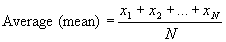Whenever possible, repeat a measurement several times and average the results. This average is the best estimate of the "true" value. The more repetitions you make of a measurement, the better this estimate will be.   Consider, as another example, the measurement of the width of a piece of paper using a meter stick. Being careful to keep the meter stick parallel to the edge of the paper (to avoid a systematic error which would cause the measured value to be consistently higher than the correct value), the width of the paper is measured at a number of points on the sheet, and the values obtained are entered in a data table. Note that the last digit is only a rough estimate, since it is difficult to read a meter stick to the nearest tenth of a millimeter (0.01 cm)
Observation Width (cm)
#1    31.33
#2    31.15
#3    31.26
#4    31.02
#5    31.20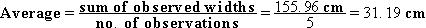This average is the best available estimate of the width of the piece of paper, but it is certainly not exact. We would have to average an infinite number of measurements to approach the true mean value, and even then, we are not guaranteed that the mean value is accurate because there is still some systematic error from the measuring tool, which can never be calibrated perfectly. So how do we express the uncertainty in our average value?

One way to express the variation among the measurements is to use the average deviation This statistic tells us on average (with 50% confidence) how much the individual measurements vary from the mean.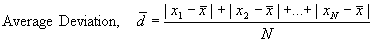However, the standard deviation is the most common way to characterize the spread of a data set. The standard deviation is always slightly greater than the average deviation, and is used because of its association with the normal distribution that is frequently encountered in statistical analyses.

Standard Deviation

To calculate the standard deviation for a sample of 5 (or more generally N) measurements:

1. Sum all the measurements and divide by 5 to get the average or mean.

2. Now, subtract this average from each of the 5 measurements to obtain 5 "deviations".

3. Square each of these 5 deviations and add them all up.

4. Divide this result by (N-1), and take the square root.

We can write out the formula for the standard deviation as follows. Let the N measurements be called x1, x2,..., xN. Let the average of the N values be called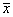. Then each deviation is given by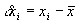, for i = 1, 2,...,N.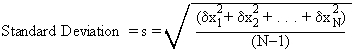or in shorter form,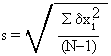In our previous example, the average widthis 31.19 cm. The deviations are:

 Observation Width (cm) Deviation (cm) #1 31.33 +0.14 = 31.33 - 31.19 #2 31.15 -0.04 = 31.15 - 31.19 #3 31.26 +0.07 = 31.26 - 31.19 #4 31.02 -0.17 = 31.02 - 31.19 #5 31.20 +0.01 = 31.20 - 31.19
The average deviation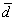= 0.086 cm
The standard deviation is: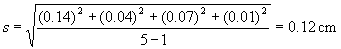The significance of the standard deviation is this: if you now make one more measurement using the same meter stick, you can reasonably expect (with about 68% confidence) that the new measurement will be within 0.12 cm of the estimated average of 31.19 cm. In fact, it is reasonable to use the standard deviation as the uncertainty associated with this single new measurement. However, the uncertainty of the average value is the standard deviation of the mean, which is always less than the standard deviation.

Consider an example where 100 measurements of a quantity were made. The average or mean value was 10.5 and the standard deviation was s = 1.83. The figure below is a histogram of the 100 measurements, which shows how often a certain range of values was measured. For example, in 20 of the measurements, the value was in the range 9.5 to 10.5, and most of the readings were close to the mean value of 10.5. The standard deviation s for this set of measurements is roughly how far from the average value most of the readings fell. For a large enough sample, approximately 68% of the readings will be within one standard deviation of the mean value, 95% of the readings will be in the interval±2s, and nearly all (99.7%) of readings will lie within 3 standard deviations from the mean. The smooth curve superimposed on the histogram is the gaussian or normal distribution predicted by theory for measurements involving random errors. As more and more measurements are made, the histogram will more closely follow the bell-shaped gaussian curve, but the standard deviation of the distribution will remain approximately the same.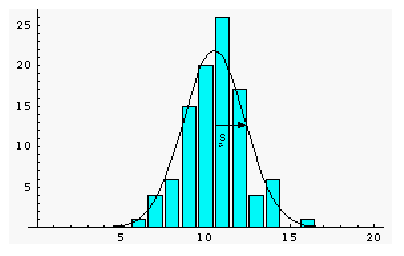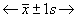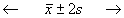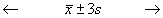Standard Deviation of the Mean (Standard Error)

When we report the average value of N measurements, the uncertainty we should associate with this average value is the standard deviation of the mean, often called the standard error (SE).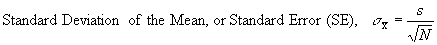(3) The standard error is smaller than the standard deviation by a factor of. This reflects the fact that we expect the uncertainty of the average value to get smaller when we use a larger number of measurements N. In the previous example, we find the standard error is 0.05 cm, where we have divided the standard deviation of 0.12 by Ö 5. The final result should then be reported as:
Average paper width = 31.19 ± 0.05 cm

### Anomalous Data

The first step you should take in analyzing data (and even while taking data) is to examine the data set as a whole to look for patterns and outliers. Anomalous data points that lie outside the general trend of the data may suggest an interesting phenomenon that could lead to a new discovery, or they may simply be the result of a mistake or random fluctuations. In any case, an outlier requires closer examination to determine the cause of the unexpected result. Extreme data should never be "thrown out" without clear justification and explanation, because you may be discarding the most significant part of the investigation! However, if you can clearly justify omitting an inconsistent data point, then you should exclude the outlier from your analysis so that the average value is not skewed from the "true" mean.

Fractional Uncertainty Revisited

When a reported value is determined by taking the average of a set of independent readings, the fractional uncertainty is given by the ratio of the uncertainty divided by the average value. For this example,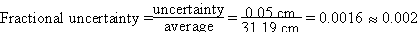Note that the fractional uncertainty is dimensionless (the uncertainty in cm was divided by the average in cm). An experimental physicist might make the statement that this measurement "is good to about 1 part in 500" or "precise to about 0.2%".

The fractional uncertainty is also important because it is used in propagating uncertainty in calculations using the result of a measurement, as discussed in the next section.

Propagation of Uncertainty

Suppose we want to determine a quantity f which depends on x, and maybe several other variables y, z, ... etc. We want to know the error in f if we measure x, y, ... with error sx, sy, ... .

Examples: f = xy ( Area of a rectangle )
f = pcosq ( x-component of momentum )

f = x / t ( velocity )

For a single-variable function f(x), the deviation in f can be related to the deviation in x using calculus: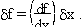Thus, taking the square and the average: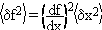,

and using the definition of s, we get: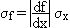.

Examples: (a) f = x2 .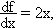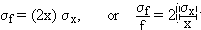i.e. the fractional error of x2 is twice the fractional error of x.

(b) f = cosq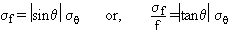Note: in this situation, sq must be in radians

In the case where f depends on two or more variables, the derivation above can be repeated with minor modification. For two variables, f(x, y), we have: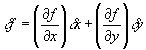The partial derivative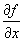means differentiating f with respect to x holding the other variables fixed.

Thus taking the square and the average, we get the law of propagation of uncertainty: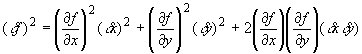(4) If the measurements of x and y are uncorrelated, then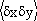= 0, and using the definition of s, we get:Examples:
(a) f = x + y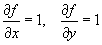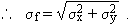(b) f = xy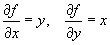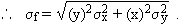Dividing the above equation by f = xy, we get :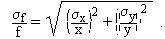(c) f = x / y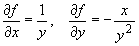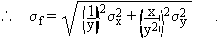Dividing the above equation by f = x / y, we get:Note that the relative uncertainty in f, as shown in (b) and (c) above, has the same form for multiplication and division: The relative uncertainty in a product or quotient is the square root of the sum of the squares of the relative uncertainty of each individual term, as long as the terms are not correlated. Example: Find uncertainty in v, where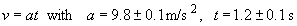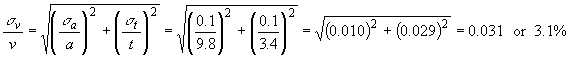Notice that since the relative uncertainty in t (2.9%) is significantly greater than the relative uncertainty for a (1.0%), the relative uncertainty in v is essentially the same as for t (about 3%).

Time-saving approximation: "A chain is only as strong as its weakest link."

If one of the uncertainty terms is more than 3 times greater than the other terms, the root-squares formula can be skipped, and the combined uncertainty is simply the largest uncertainty. This shortcut can save a lot of time without losing any accuracy in the estimate of the overall uncertainty. The Upper-Lower Bound Method of Uncertainty Propagation

An alternative and sometimes simpler procedure to the tedious propagation of uncertainty law that is the upper-lower bound method of uncertainty propagation. This altermative method does not yield a standard uncertainty estimate (with a 68% confidence interval), but it does give a reasonable estimate of the uncertainty for practically any situation. The basic idea of this method is to use the uncertainty ranges of each variable to calculate the maximum and minimum values of the function. You can also think of this procedure as examining the best and worst case scenarios. For example, if you took an angle measurement: q = 25°± 1° and you needed to find f = cosq , then

fmax = cos(26° ) = 0.8988
fmin = cos(24° ) = 0.9135 \f » 0.906 ±0.007 Note that even though q was only measured to 2 significant figures, f is known to 3 figures.

By using the propagation of uncertainty law: sf = |sinq |sq = (0.423)(1/180) = 0.0023

As shown in this example,

The uncertainty estimate from the upper-lower bound method is generally larger than the standard uncertainty estimate found from the propagation of uncertainty law. The upper-lower bound method is especially useful when the functional relationship is not clear or is incomplete. One practical application is forecasting the expected range in an expense budget. In this case, some expenses may be fixed, while others may be uncertain, and the range of these uncertain terms could be used to predict the upper and lower bounds on the total expense.

Significant Figures

The number of significant figures in a value can be defined as all the digits between and including the first non-zero digit from the left, through the last digit.

For instance, 0.44 has two significant figures, and the number 66.770 has 5 significant figures. Zeroes are significant except when used to locate the decimal point, as in the number 0.00030, which has 2 significant figures. Zeroes may or may not be significant for numbers like 1200, where it is not clear whether two, three, or four significant figures are indicated. To avoid this ambiguity, such numbers should be expressed in scientific notation to (e.g. 1.2 x 103 clearly indicates two significant figures).

When using a calculator, the display will often show many digits, only some of which are meaningful (significant in a different sense). For example, if you want to estimate the area of a circular playing field, you might pace off the radius to be 9 meters and use the formula area = pr2. When you compute this area, the calculator might report a value of 254.4690049 m2. It would be extremely misleading to report this number as the area of the field, because it would suggest that you know the area to an absurd degree of precision - to within a fraction of a square millimeter! Since the radius is only known to one significant figure, the final answer should also contain only one significant figure. A more truthful answer would be to report the area as 300 m2; however, this format is somewhat misleading, since it could be interpreted to have three significant figures because of the zeroes. The better way to report the number would be to use scientific notation: 3 ´ 102 m2.

From this example, we can see that the number of significant figures reported for a value implies a certain degree of precision. In fact, the number of significant figures suggests a rough estimate of the relative uncertainty:

The number of significant figures implies an approximate relative uncertainty
1 significant figure suggests a relative uncertainty of about 10% to 100%
2 significant figures suggest a relative uncertainty of about 1% to 10%
3 significant figures suggest a relative uncertainty of about 0.1% to 1%
To understand this connection more clearly, consider a value with 2 significant figures, like 99, which suggests an uncertainty of ± 1, or a relative uncertainty of ± 1%. (Actually some people might argue that the implied uncertainty in 99 is ± 0.5 since the range of values that would round to 99 are 98.5 to 99.4. But since the uncertainty here is only a rough estimate, there is not much point arguing about the factor of 2 difference.) The smallest 2-significant figure number, 10, also suggests an uncertainty of ± 1, which in this case is a relative uncertainty of ± 10%. The ranges for other numbers of significant figures can be reasoned in a similar manner.

Use of Significant Figures for Simple Propagation of Uncertainty

By following a few simple rules, significant figures can be used to find the appropriate precision for a calculated result for the four most basic math functions, all without the use of complicated formulas for propagating uncertainties.

For multiplication and division, the number of significant figures that are reliably known in a product or quotient is the same as the smallest number of significant figures in any of the original numbers.
Example:         6.6 (2 significant figures)
x 7328.7 (5 significant figures)
48369.42 = 48 x 103 (2 significant figures)
For addition and subtraction, the result should be rounded off to the last decimal place reported for the least precise number.
Examples: 223.64    5560.5
+54            +0.008
278         5560.5

If a calculated number is to be used in further calculations, it is good practice to keep one extra digit to reduce rounding errors that may accumulate. Then the final answer should be rounded according to the above guidelines.
Uncertainty and Significant Figures

For the same reason that it is dishonest to report a result with more significant figures than are reliably known, the uncertainty value should also not be reported with excessive precision.

For example, if we measure the density of copper, it would be unreasonable to report a result like:

measured density = 8.93 ± 0.4753 g/cm3 WRONG!
The uncertainty in the measurement cannot be known to that precision. In most experimental work, the confidence in the uncertainty estimate is not much better than about ± 50% because of all the various sources of error, none of which can be known exactly. Therefore, to be consistent with this large uncertainty in the uncertainty (!) the uncertainty value should be stated to only one significant figure (or perhaps 2 sig. figs. if the first digit is a 1).
Experimental uncertainties should be rounded to one (or at most two) significant figures.
To help give a sense of the amount of confidence that can be placed in the standard deviation, the following table indicates the relative uncertainty associated with the standard deviation for various sample sizes. Note that in order for an uncertainty value to be reported to 3 significant figures, more than 10,000 readings would be required to justify this degree of precision!

 N Relative Uncert.* Sig.Figs. Valid Implied Uncertainty 2 71% 1 ± 10% to 100% 3 50% 1 ± 10% to 100% 4 41% 1 ± 10% to 100% 5 35% 1 ± 10% to 100% 10 24% 1 ± 10% to 100% 20 16% 1 ± 10% to 100% 30 13% 1 ± 10% to 100% 50 10% 2 ± 1% to 10% 100 7% 2 ± 1% to 10% 10000 0.7% 3 ± 0.1% to 1%

*The relative uncertainty is given by the approximate formula: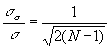When an explicit uncertainty estimate is made, the uncertainty term indicates how many significant figures should be reported in the measured value (not the other way around!). For example, the uncertainty in the density measurement above is about 0.5 g/cm3, so this tells us that the digit in the tenths place is uncertain, and should be the last one reported. The other digits in the hundredths place and beyond are insignificant, and should not be reported:

measured density = 8.9 ± 0.5 g/cm3 RIGHT!
An experimental value should be rounded to an appropriate number of significant figures consistent with its uncertainty. This generally means that the last significant figure in any reported measurement should be in the same decimal place as the uncertainty.

In most instances, this practice of rounding an experimental result to be consistent with the uncertainty estimate gives the same number of significant figures as the rules discussed earlier for simple propagation of uncertainties for adding, subtracting, multiplying, and dividing.

Caution: When conducting an experiment, it is important to keep in mind that precision is expensive (both in terms of time and material resources). Do not waste your time trying to obtain a precise result when only a rough estimate is require. The cost increases exponentially with the amount of precision required, so the potential benefit of this precision must be weighed against the extra cost.

Combining and Reporting Uncertainties

In 1993, the International Standards Organization (ISO) published the first official world-wide Guide to the Expression of Uncertainty in Measurement. Before this time, uncertainty estimates were evaluated and reported according to different conventions depending on the context of the measurement or the scientific discipline. Here are a few key points from this 100-page guide, which can be found in modified form on the NIST website (see References).

When reporting a measurement, the measured value should be reported along with an estimate of the total combined standard uncertainty of the value. The total uncertainty is found by combining the uncertainty components based on the two types of uncertainty analysis:

Type A evaluation of standard uncertainty  method of evaluation of uncertainty by the statistical analysis of a series of observations. This method primarily includes random errors.

Type B evaluation of standard uncertainty  method of evaluation of uncertainty by means other than the statistical analysis of series of observations. This method includes systematic errors and any other uncertainty factors that the experimenter believes are important.

The individual uncertainty components should be combined using the law of propagation of uncertainties, commonly called the "root-sum-of-squares" or "RSS" method. When this is done, the combined standard uncertainty should be equivalent to the standard deviation of the result, making this uncertainty value correspond with a 68% confidence interval. If a wider confidence interval is desired, the uncertainty can be multiplied by a coverage factor (usually k = 2 or 3) to provide an uncertainty range that is believed to include the true value with a confidence of 95% or 99.7% respectively. If a coverage factor is used, there should be a clear explanation of its meaning so there is no confusion for readers interpreting the significance of the uncertainty value.

You should be aware that the ± uncertainty notation may be used to indicate different confidence intervals, depending on the scientific discipline or context. For example, a public opinion poll may report that the results have a margin of error of ± 3%, which means that readers can be 95% confident (not 68% confident) that the reported results are accurate within 3 percentage points. In physics, the same average result would be reported with an uncertainty of ± 1.5% to indicate the 68% confidence interval.

Conclusion: "When do measurements agree with each other?"

We now have the resources to answer the fundamental scientific question that was asked at the beginning of this error analysis discussion: "Does my result agree with a theoretical prediction or results from other experiments?"

Generally speaking, a measured result agrees with a theoretical prediction if the prediction lies within the range of experimental uncertainty. Similarly, if two measured values have standard uncertainty ranges that overlap, then the measurements are said to be consistent (they agree). If the uncertainty ranges do not overlap, then the measurements are said to be discrepant (they do not agree). However, you should recognize that this overlap criteria can give two opposite answers depending on the evaluation and confidence level of the uncertainty. It would be unethical to arbitrarily inflate the uncertainty range just to make the measurement agree with an expected value. A better procedure would be to discuss the size of the difference between the measured and expected values within the context of the uncertainty, and try to discover the source of the discrepancy if the difference is truly significant.

References:

Taylor, John. An Introduction to Error Analysis, 2nd. ed. University Science Books: Sausalito, 1997.

Baird, D.C. Experimentation: An Introduction to Measurement Theory and Experiment Design, 3rd. ed. Prentice Hall: Englewood Cliffs, 1995.

Bevington, Phillip and Robinson, D. Data Reduction and Error Analysis for the Physical Sciences, 2nd. ed. McGraw-Hill: New York, 1991.

ISO. Guide to the Expression of Uncertainty in Measurement. International Organization for Standardization (ISO) and the International Committee on Weights and Measures (CIPM): Switzerland, 1993.

NIST. Guidelines for Evaluating and Expressing the Uncertainty of NIST Measurement Results, 1994. Available online: http://physics.nist.gov/Pubs/guidelines/contents.html

Copyright:  The University of North Carolina at Chapel Hill, Department of Physics and Astronomy
Last revised:  August 10, 2000 by Duane Deardorff, Director of Undergraduate Laboratories